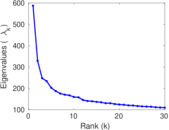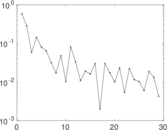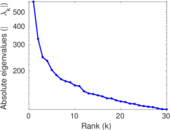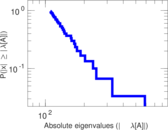# Flickr

This is the bipartite network of Flickr users and their group memberships.

 Code `FG` Internal name `flickr-groupmemberships` Name Flickr Data source http://socialnetworks.mpi-sws.org/data-imc2007.html AvailabilityDataset is available for download Consistency checkDataset passed all tests Category Affiliation network Dataset timestamp 2007 Node meaning User, group Edge meaning Membership Network formatBipartite, undirected Edge typeUnweighted, no multiple edges

## Statistics

 Size n = 499,610 Left size n1 = 395,979 Right size n2 = 103,631 Volume m = 8,545,307 Wedge count s = 9,755,612,929 Claw count z = 34,392,804,882,987 Cross count x = 183,897,960,553,802,528 Square count q = 35,258,013,095 4-Tour count T4 = 321,104,865,090 Maximum degree dmax = 34,989 Maximum left degree d1max = 2,186 Maximum right degree d2max = 34,989 Average degree d = 34.207 9 Average left degree d1 = 21.580 2 Average right degree d2 = 82.459 0 Fill p = 0.000 208 241 Size of LCC N = 470,345 Diameter δ = 18 50-Percentile effective diameter δ0.5 = 3.720 57 90-Percentile effective diameter δ0.9 = 5.622 14 Median distance δM = 4 Mean distance δm = 4.437 56 Gini coefficient G = 0.849 060 Balanced inequality ratio P = 0.144 240 Left balanced inequality ratio P1 = 0.178 314 Right balanced inequality ratio P2 = 0.146 343 Relative edge distribution entropy Her = 0.858 658 Power law exponent γ = 1.583 20 Tail power law exponent γt = 1.451 00 Degree assortativity ρ = −0.052 003 7 Degree assortativity p-value pρ = 0.000 00 Spectral norm α = 587.208 Spectral separation |λ1[A] / λ2[A]| = 1.784 40

## Plots

### Degree distribution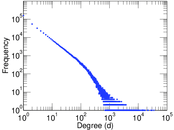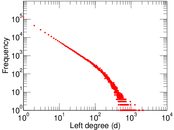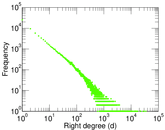### Cumulative degree distribution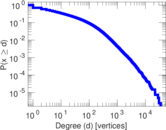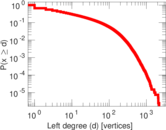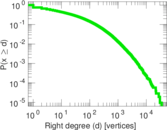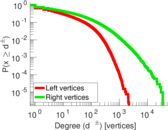### Lorenz curve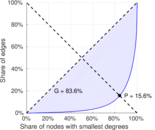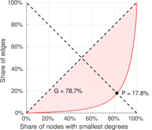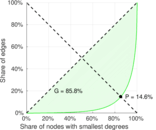### Spectral distribution of the adjacency matrix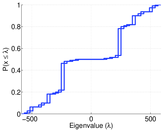### Spectral distribution of the normalized adjacency matrix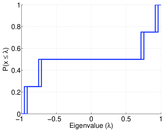### Spectral distribution of the Laplacian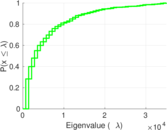### Spectral graph drawing based on the adjacency matrix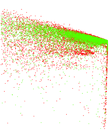### Spectral graph drawing based on the Laplacian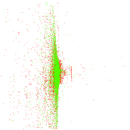### Spectral graph drawing based on the normalized adjacency matrix### Degree assortativity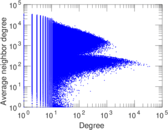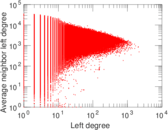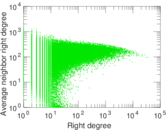### Zipf plot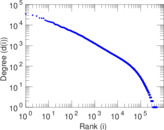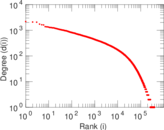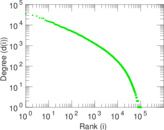### Hop distribution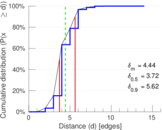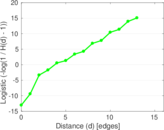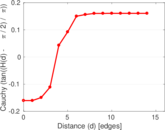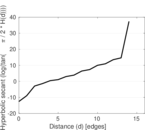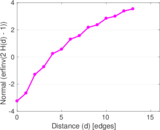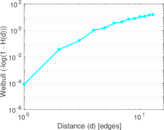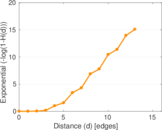### Delaunay graph drawing### Matrix decompositions plots